Decimal Numbers

Chapter 2 Class 7 Fractions and Decimals
Concept wise

Decimal are number which have a decimal point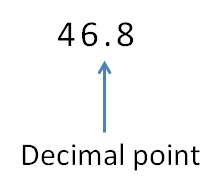Decimal number are number between two whole numbers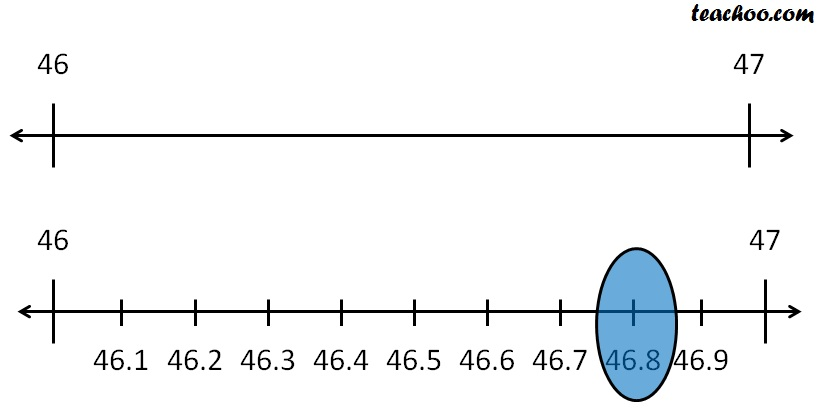But, what does decimal point mean ?

Let ‘s look at place value first

#### Place value of numbers with decimal point

For number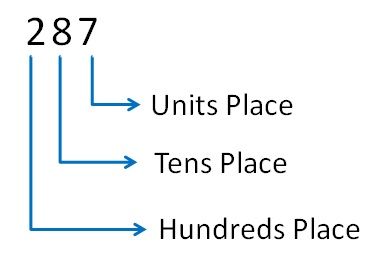The numbers  is

Two hundred eighty seven

287 = 2 × 100 + 8 × 10  + 7

So,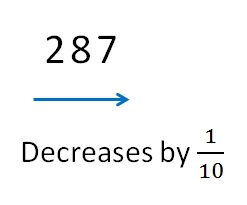Suppose we put a decimal point as well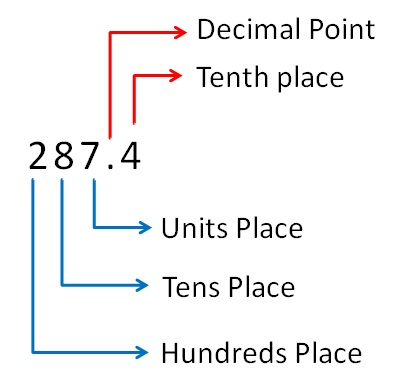The numbers  is

Two hundred eighty seven point four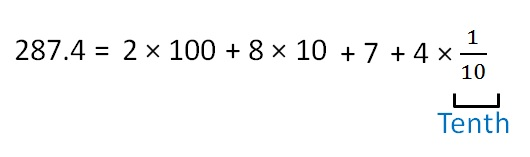Similarly,

We can have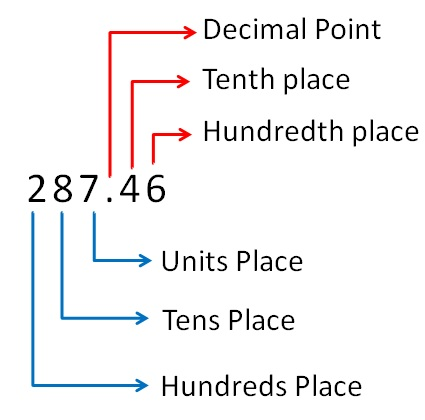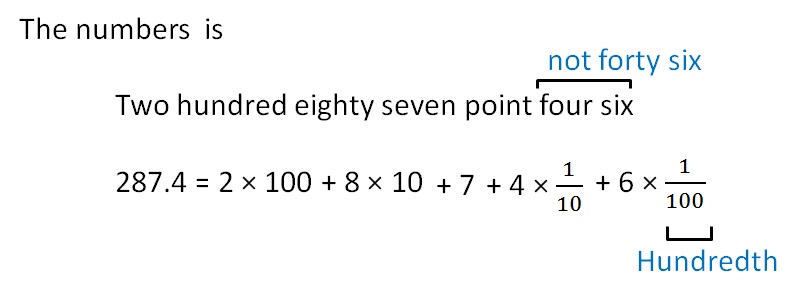Introducing your new favourite teacher - Teachoo Black, at only ₹83 per month

### Transcript

Similarly, We can have The numbers is Two hundred eighty seven point four six 287.4 = Decimal Point Tenth place Hundredth place